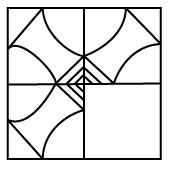## SSC CGL Reasoning Test 12

Instructions

In each of the following questions, which answer figure
will complete the pattern in the question figure ?

Question 1Question 2Instructions

For the following questions answer them individually

Question 3

If PKROK is coded as 72962 and KRRPK as 29972 then how can NJMLZ be coded ?

Question 4

If 1 + 4=9, 2 + 8=18, 3+6 = 15 then 7 + 8 = ?

Question 5

$$\frac{15}{20}+\frac{4}{5}\times\frac{2}{3}\times\frac{8}{5}=?$$